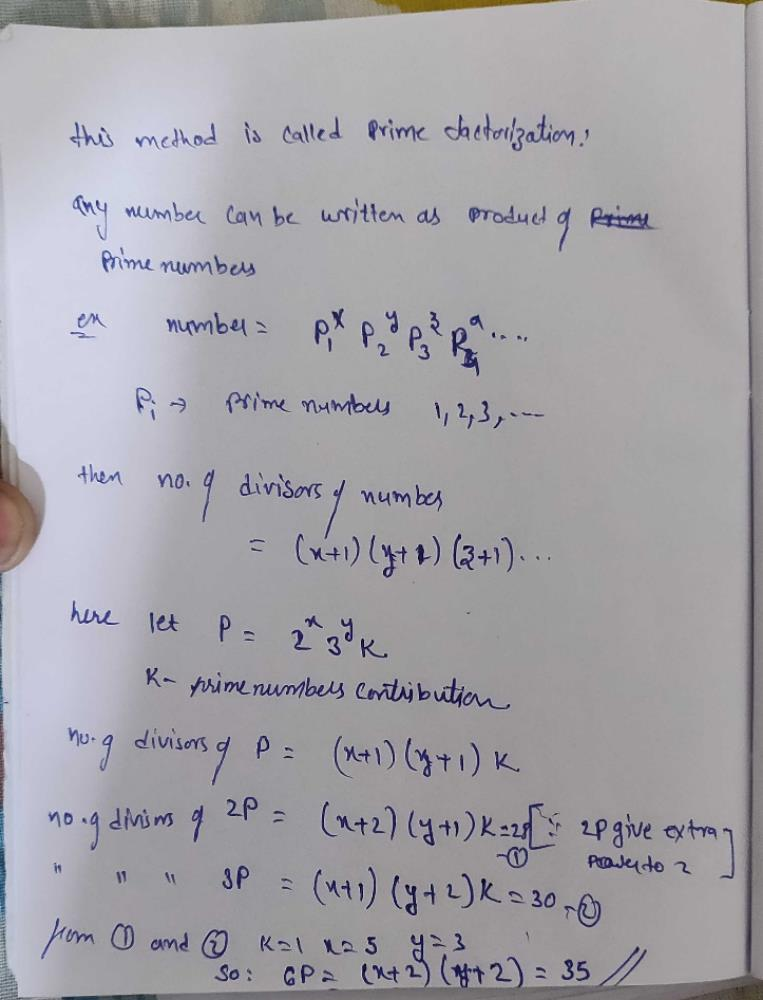Courses

# P is a natural number. 2P has 28 divisors and 3P has 30 divisors. How many divisors of 6P will be there?a)35    b)40    c)45    d)48Correct answer is option 'A'. Can you explain this answer?

## Quant QuestionDinesh Kukreja Jun 11, 2018
Solution 1:
2P is having 28(4*7) divisors but 3P is not having a total divisors which is divisible by 7, so the first part of the number P will be 2^5.
Similarly, 3P is having 30 (3*10) divisors but 2P does not have a total divisors which is divisible by 3. So 2nd part of the number P will be 3^3. So, P = 2^5*3^3 and the solution is 35.

Solution 2:
2P has 28 divisors =4x7,
3P has 30 divisors
Hence P=2^5 3^3
6p =2^6 3^4
Hence 35 divisorsRahul Prajapati Aug 13, 2019
Solution doesn't satisfie, can someone solve this correctly.Dhanaraj S Jul 25, 2020
Coming to concepts of prime factorization

any number can be expressed as powers of products of prime numbers ,
also the thing to note is that, the number of divisors of a number thus can be obtained by this prime factorization, which is product of (powers+1)
let a=2^a*3^b....
so no of divisor =( a+1)(b+1)....

here let
p has r divisor
r=(a+1)(b+1)k
28=(a+2)(b+1)k. where k is contribution from other primes than 2 and 3

similarly,
30 =(a+1)(b+2)k
by trial and error
28=1*4*7
30=1*6*5
thus x=5
y =3
k=1
so 3p has (a+2)(b+2)k divisor
=35 divisorRaghav Sharma Feb 14, 2020